[top.htm]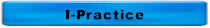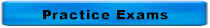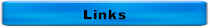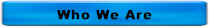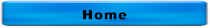Answers for More Math Problems & Charts

The controls for a pump are set for the pump to turn on when the pressure drops to 2 psi and to turn off when the pressure reaches 8 psi.  If this tank has a diameter of 50 feet, how many gallons will be stored between pumping cycles?

Diameter = 50 feet and from the solution below, depth = 13.86 ft

Volume, gallons = (0.785)(d2)(depth, ft)(7.48 gal/ft3) = (0.785)(502)(13.86)(7.48) = 203,458 gallons

The controls for a pump are set for the pump to turn on when the pressure drops to 2 psi and to turn off when the pressure reaches 8 psi.  Calculate the following items:

1.  The depth of liquid when the pump turns on

(2 psi)(2.31 ft/psi) = 4.62 ft

2.  The depth of the liquid when the pump turns off

(8 psi)(2.31 ft/psi) = 18.48 ft

3.  The depth of the liquid that can be pumped

18.48 ft - 4.62 ft = 13.86 ft

A centrifugal pump delivering 200 gpm against a total head of 40 feet produces _______ HP

WHP = (Flow, gpm)(Head, ft) � 3,960 = (200 gpm)(40 ft) � 3,960 = 2 WHP

A lot which measures 1200 feet by 1500 feet contains ______ acres

(1200 feet)(1500 ft) = 1,800,000 square feet

(1,800,000 square feet) � (43,560 sf/acre) = 41.3 acres

3 cubic yards contains _____ cubic feet

(3 cubic yards)(27 cubic feet/cubic yard) = 81 cubic feet

1 yard = 3 feet

1 cubic yard = (1 yard)(1 yard)(1 yard) = (3 ft)(3 ft)(3 ft) = 27 cubic feet

Calculate the pumping rate for a pump if the wet well drops 4 feet in 30 minutes. The wet well is 12 feet wide and 15 feet long.

Volume, cf = (Length, ft)(Width, ft)(Depth, ft) = (15 ft)(12 ft)(4 ft) = 720 cf

Capacity, gal = (Volume, cf)(7.48 gal/cf) = 5,385.6 gal

Flow, gpm = (Capacity, gal) � (Time, min) = 5,385.6 gal � 30 minutes = 180 gpm

If you dig a trench 500 feet long, 3 feet wide, and 8 feet deep, how many cubic yards of spoil will you remove?

Volume, cf = (Length, ft)(Width, ft)(Depth, ft) = (500 ft)(3 ft)(8 ft) = 12,000 cf

Volume, cy = (Volume, cf) � (27 cf/cy) = 444 cy

What is the discharge pressure of your pump if you're pumping into a force main that is 40 feet above the pump and you need to maintain a pressure of 60 psi in the force main?

Have you tried using my box converter to solve this type of problem?

 1 psi 2.31 ft 40 ft

40 ft � 2.31 ft/psi = 17.3 psi

Discharge pressure = 60 psi + 17.3 psi = 77.3 psi

If the discharge pressure gauge reads 80 psi, what is the equivalent head in feet?

Have you tried using my box converter to solve this type of problem?

 1 psi 80 psi 2.31 ft

2.31 ft/psi x 80 psi = 184.8 ft

Calculate the flow in cfs for a 6-ft wide channel if the flow is 18 inches deep and the velocity is 2 fps,

Flow Rate, cfs = (Cross-sectional Area, sf) (Velocity, ft/sec)

Cross-sectional Area, sf = (Length, ft) (Depth, ft)

Depth, ft = Depth, in � 12 in/ft = 18 in � 12 in/ft = 1.5 sf

Cross-sectional Area, sf = (Length, ft) (Depth, ft) = (6 ft)(1.5 ft) = 9 sf

Flow Rate, cfs = (Cross-sectional Area, sf) (Velocity, ft/sec) = (9 sf)(2 fps) = 18 cfs

6 mgd = _________ cfs

6 mgd x 1.55 cfs/mgd = 9.3 cfs

The temperature of your sample is 16oC.  What is this in oF?

oF = (oC)(1.8) + 32 = (16oC)(1.8) + 32 = 28.8 + 32 = 60.8oF

If you thicken your solids from 2% to 5% and you typically pump 1000 gallons per day of 2%, how many gallons of 5% solids will you pump?

(C1)(V1) = (C2)(V2)

(5%)(??? gallons) = (2%)(1000 gallons)

(5%)(??? gallons) = 2000%gallons

(??? gallons) � 5% = (2000%gallons) � 5% =  400 gallons

If you thicken your solids from 4% to 6% and you typically pump 12,000 gallons per day of 4%, how many gallons of 6% solids will you pump?

(C1)(V1) = (C2)(V2)

(6%)(??? gallons) = (4%)(12,000 gallons)

(6%)(??? gallons) = 48,000%gallons

(6%)(??? gallons) � 6% = (48,000%gallons) � 6% = 8,000 gallons

A lift station is equipped with three pumps, each rated at 175 gpm.   The wet well is 20 feet long, 10 feet wide, and 9 feet deep.  The average influent flow is 0.5 mgd.

• With no influent flow, one pump will empty the wet well in _______ minutes

20 ft x 10 ft x 9 ft = 1,800 cf

1,800 cf x 7.5 gal/cf = 13,500 gal

13,500 gal � 175 gpm = 77.1 min

• With no influent flow and one pump running, the water level in the wet well will drop ________ feet in 10 minutes

10 min � 77.1 min = 0.13

0.13 x 9 ft = 1.17 ft

Given this lab data, calculate the TSS in mg/L

• Tare Weight  =  20.0012 g
• Final Dry Weight  =  20.0015 g
• Sample Volume  =  50 mL

Solids, mg/L =    (Dry Solids, grams)(1,000,000)
Sample Volume, mL

Dry Solids, g    = Final Dry Weight, g - Tare Weight, g

= 20.0015 g - 20.0012 g = 0.0003 g

Solids, mg/L =    (0.00030 g)(1,000,000)
50 mL

= 300 �  50

= 6 mg/L

Your sludge pump has one 12-inch piston with a 9-inch stroke.  It is rated at 50 RPM and is set to pump 2 minutes every 10 minutes.  The total solids concentration is 5%.  If the temperature of the raw sludge is 50oF and the digester is at 95oF, how many BTUs are required to warm the sludge?

1. Convert inches to feet

12 inch piston � 12 in/ft = 1 ft

9-inch stroke � 12 in/ft = 0.75 ft

1. Calculate the volume displaced for one stroke

(0.785)(1 ft)(1 ft)(0.75 ft)(7.48 gal/ft) = 4.4 gal/stroke

1. Calculate the gpm (RPM = strokes/min)

4.4 gal/stroke x 50 RPM = 220 gpm

1. Calculate gpd of sludge

(220 gpm)(2 min/10 min)(1440 min/day) =  63,360 gpd of sludge

1. Convert to lbs/day of sludge

(63,360 gpd)(8.34 lbs/gal) = 528,422 lbs/day of sludge

1. Calculate the temperature increase

95oF - 50oF = 45oF

1. Calculate the BTUs required

(528,422 lbs/day of sludge)(45oF) = 23,778,990 BTUs

Your sludge pump has one 12-inch piston with a 9-inch stroke.  It is rated at 50 RPM and is set to pump 2 minutes every 10 minutes.  The total solids concentration of the sludge is 5% and the volatile solids concentration is 70%.  Your digester typically produce 12 cubic feet of digester gas per pound of volatile solids fed to the digester and averages 650 BTU/cubic foot of digester gas, how many BTUs should be produced?

1. Convert inches to feet

12 inch piston � 12 in/ft = 1 ft

9-inch stroke � 12 in/ft = 0.75 ft

1. Calculate the volume displaced for one stroke

(0.785)(1 ft)(1 ft)(0.75 ft)(7.48 gal/ft) = 4.4 gal/stroke

1. Calculate the gpm (RPM = strokes/min)

4.4 gal/stroke x 50 RPM = 220 gpm

1. Calculate gpd of sludge

(220 gpm)(2 min/10 min)(1440 min/day) =  63,360 gpd of sludge

1. Convert to lbs/day of sludge

(63,360 gpd)(8.34 lbs/gal) = 528,422 lbs/day of sludge

1. Convert %Total Solids (TS) to decimal equivalent

5% TS � 100 = 0.05 TS

1. Calculate lbs/day of Total Solids

(528,422 lbs/day of sludge)(0.05 TS) = 26,421 lbs/day of TS

1. Calculate lbs/day of Volatile Solids

(26,421 lbs/day of TS)(0.70 VS) = 18,495 lbs/day of VS

1. Calculate lbs/day of cubic feet of digester gas produced

(18,495 lbs/day of VS)(12 cf/lb) = 221,940 cubic of digester gas produced

1. Calculate the BTUs produced

(221,940 cf)(650 BTU/cf) = 14,261,000 BTU

OR

1. Convert to MGD of sludge

(63,360 gpd) (1,000,000 gpd/MGD) = 0.06336 MGD

1. Convert %Total Solids (TS) to mg/L

(5% TS)(10,000 mg/L/%)= 50,000 mg/L TS

1. Calculate lbs/day of Total Solids

(50,000 mg/L)(8.34 lbs/gal)(0.06336 MGD) = 26,421 lbs/day of TS

1. Calculate lbs/day of Volatile Solids

(26,421 lbs/day of TS)(0.70 VS) = 18,495 lbs/day of VS

1. Calculate lbs/day of cubic feet of digester gas produced

(18,495 lbs/day of VS)(12 cf/lb) = 221,940 cubic of digester gas produced

1. Calculate the BTUs produced

(221,940 cf)(650 BTU/cf) = 14,261,000 BTU

Your sludge pump has one 12-inch piston with a 9-inch stroke.  It is rated at 50 RPM and is set to pump 2 minutes every 10 minutes.  The total solids concentration of the sludge is 5% and the volatile solids concentration is 70%.  If your digester typically produce 12 cubic feet of digester gas per pound of volatile solids fed to the digester, how many cubic feet of digester gas should be produced?

1. Convert inches to feet

12 inch piston � 12 in/ft = 1 ft

9-inch stroke � 12 in/ft = 0.75 ft

1. Calculate the volume displaced for one stroke

(0.785)(1 ft)(1 ft)(0.75 ft)(7.48 gal/ft) = 4.4 gal/stroke

1. Calculate the gpm (RPM = strokes/min)

4.4 gal/stroke x 50 RPM = 220 gpm

1. Calculate gpd of sludge

(220 gpm)(2 min/10 min)(1440 min/day) =  63,360 gpd of sludge

1. Convert to lbs/day of sludge

(63,360 gpd)(8.34 lbs/gal) = 528,422 lbs/day of sludge

1. Convert %Total Solids (TS) to decimal equivalent

5% TS � 100 = 0.05 TS

1. Calculate lbs/day of Total Solids

(528,422 lbs/day of sludge)(0.05 TS) = 26,421 lbs/day of TS

1. Calculate lbs/day of Volatile Solids

(26,421 lbs/day of TS)(0.70 VS) = 18,495 lbs/day of VS

1. Calculate lbs/day of cubic feet of digester gas produced

(18,495 lbs/day of VS)(12 cf/lb) = 221,940 cubic of digester gas produced

OR

1. Convert to MGD of sludge

(63,360 gpd) (1,000,000 gpd/MGD) = 0.06336 MGD

1. Convert %Total Solids (TS) to mg/L

(5% TS)(10,000 mg/L/%)= 50,000 mg/L TS

1. Calculate lbs/day of Total Solids

(50,000 mg/L)(8.34 lbs/gal)(0.06336 MGD) = 26,421 lbs/day of TS

1. Calculate lbs/day of Volatile Solids

(26,421 lbs/day of TS)(0.70 VS) = 18,495 lbs/day of VS

1. Calculate lbs/day of cubic feet of digester gas produced

(18,495 lbs/day of VS)(12 cf/lb) = 221,940 cubic of digester gas produced

Your sludge pump has one 12-inch piston with a 9-inch stroke.  It is rated at 50 RPM and is set to pump 2 minutes every 10 minutes.  The total solids concentration of the sludge is 5% and the volatile solids concentration is 70%, how many pounds of volatile solids are pumped daily?

1. Convert inches to feet

12 inch piston � 12 in/ft = 1 ft

9-inch stroke � 12 in/ft = 0.75 ft

1. Calculate the volume displaced for one stroke

(0.785)(1 ft)(1 ft)(0.75 ft)(7.48 gal/ft) = 4.4 gal/stroke

1. Calculate the gpm (RPM = strokes/min)

4.4 gal/stroke x 50 RPM = 220 gpm

1. Calculate gpd of sludge

(220 gpm)(2 min/10 min)(1440 min/day) =  63,360 gpd of sludge

1. Convert to lbs/day of sludge

(63,360 gpd)(8.34 lbs/gal) = 528,422 lbs/day of sludge

1. Convert %Total Solids (TS) to decimal equivalent

5% TS � 100 = 0.05 TS

1. Calculate lbs/day of Total Solids

(528,422 lbs/day of sludge)(0.05 TS) = 26,421 lbs/day of TS

1. Calculate lbs/day of Volatile Solids

(26,421 lbs/day of TS)(0.70 VS) = 18,495 lbs/day of VS

OR

1. Convert to MGD of sludge

(63,360 gpd) (1,000,000 gpd/MGD) = 0.06336 MGD

1. Convert %Total Solids (TS) to mg/L

(5% TS)(10,000 mg/L/%)= 50,000 mg/L TS

1. Calculate lbs/day of Total Solids

(50,000 mg/L)(8.34 lbs/gal)(0.06336 MGD) = 26,421 lbs/day of TS

1. Calculate lbs/day of Volatile Solids

(26,421 lbs/day of TS)(0.70 VS) = 18,495 lbs/day of VS

Your sludge pump has one 12-inch piston with a 9-inch stroke.  It is rated at 50 RPM and is set to pump 2 minutes every 10 minutes.  If the total solids concentration is 5%, how many pounds of total solids are pumped daily?

1. Convert inches to feet

12 inch piston � 12 in/ft = 1 ft

9-inch stroke � 12 in/ft = 0.75 ft

1. Calculate the volume displaced for one stroke

(0.785)(1 ft)(1 ft)(0.75 ft)(7.48 gal/ft) = 4.4 gal/stroke

1. Calculate the gpm (RPM = strokes/min)

4.4 gal/stroke x 50 RPM = 220 gpm

1. Calculate gpd of sludge

(220 gpm)(2 min/10 min)(1440 min/day) =  63,360 gpd of sludge

1. Convert to lbs/day of sludge

(63,360 gpd)(8.34 lbs/gal) = 528,422 lbs/day of sludge

1. Convert %Total Solids (TS) to decimal equivalent

5% TS � 100 = 0.05 TS

1. Calculate lbs/day of Total Solids

(528,422 lbs/day of sludge)(0.05 TS) = 26,421 lbs/day of TS

OR

1. Convert to MGD of sludge

(63,360 gpd) (1,000,000 gpd/MGD) = 0.06336 MGD

1. Convert %Total Solids (TS) to mg/L

(5% TS)(10,000 mg/L/%)= 50,000 mg/L TS

1. Calculate lbs/day of Total Solids

(50,000 mg/L)(8.34 lbs/gal)(0.06336 MGD) = 26,421 lbs/day of TS

Your sludge pump has one 12-inch piston with a 9-inch stroke.  It is rated at 50 RPM and is set to pump 2 minutes every 10 minutes.   How many gallons of sludge are pumped daily?

1. Convert inches to feet

12 inch piston � 12 in/ft = 1 ft

9-inch stroke � 12 in/ft = 0.75 ft

1. Calculate the volume displaced for one stroke

(0.785)(1 ft)(1 ft)(0.75 ft)(7.48 gal/ft) = 4.4 gal/stroke

1. Calculate the gpm (RPM = strokes/min)

4.4 gal/stroke x 50 RPM = 220 gpm

1. Calculate gpd

(220 gpm)(2 min/10 min)(1440 min/day) =  63,360 gpd

If a pump has one 12-inch piston with a 9-inch stroke, and is rated at 50 RPM, how many gpm will this pump deliver?

1. Convert inches to feet

12 inch piston � 12 in/ft = 1 ft

9-inch stroke � 12 in/ft = 0.75 ft

1. Calculate the volume displaced for one stroke

(0.785)(1 ft)(1 ft)(0.75 ft)(7.48 gal/ft) = 4.4 gal/stroke

1. Calculate the gpm (RPM = strokes/min)

4.4 gal/stroke x 50 RPM = 220 gpm

To determine the weight of a tank, you should:

A.  Calculate the volume in gallons and divide by 1,000,000

B.  Calculate the volume in gallons and multiply by 8.34

C.  Divide the depth by 2.31

D.  Divide the volume by the flow

The correct answer is B.  Calculate the volume and multiply by 8.34

A is incorrect.  This is how you would calculate the volume in million gallons

C is incorrect.  This is how you would calculate the pressure on the bottom of the tank

D is incorrect.  This is how you would calculate detention time.

mg/L x 8.34 x MGD is the formula for calculating

A. Chlorine demand

B. lbs

C. lbs/day

D. Volume of a cylindrical tank

The correct answer is C.  lbs/day

Click on the thumbnail below for the answers for the chart of pump station math for cylindrical tanks.

Click on the thumbnail below for the answers for the chart of pump station math for rectangular tanks.

Click on the thumbnail below for the answers for the chart of pipe math.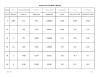Match 1 The units for pressure are A Divided by 2 Head is expressed in B 2.31 3 Velocity is measured in C 0.434 psi 4 Units of flow D psi 5 Pressure times _______ = Head E fps 6 Head __________ 2.31 = Pressure F 1440 7 20 psi = G 694 gpm 8 1 MGD = H cfs 9 A head loss of 7 feet = I 3 psi J Times K ft L 46.2 ft M Subtracted by

1 = D

2 = K

3 = E

4= H

5 = B

6 = A

7 = L

8 = G

9 = I

The formula for the volume of a rectangular tank is

A.  (Length)(Width)

B.  (0.785)(Diameter2)

C.  (Length)(Width)(Height)

D.  (0.785)(Diameter2)(Height)

The correct answer is C.  (Length)(Width)(Height)

A is the formula for the area of a rectangle

B is the formula for the area of a circle

D is the formula for the volume of a cylinder

The formula for the volume of a cylindrical tank is

A.  (Length)(Width)

B.  (0.785)(Diameter2)

C.  (Length)(Width)(Height)

D.  (0.785)(Diameter2)(Height)

The correct answer is D.  (0.785)(Diameter2)(Height)

A is the formula for the area of a rectangle

B is the formula for the area of a circle

C is the formula for the volume of a rectangle

Given a tank measures 50 feet long, 25 feet wide, and 15 ft deep, calculate:

• Surface area in ft2
• Cross-sectional area in square feet
• Volume in cf
• Capacity in gallons

The correct answers are:

• Surface area in ft2

Surface area in ft2 = Length, ft x Width, ft = 50 ft x 25 ft = 1250 ft2

• Cross-sectional area in square feet

Cross-sectional area in ft2 = Width, ft x Depth, ft = 25 ft x 15 ft = 375 square feet

• Volume in cf

Volume in cf = Length, ft x Width, ft x Depth, ft = 50 ft x 25 ft x 15 ft = 18,750 cf

• Capacity in gallons

Capacity in gallons = Vol in cf x 7.5 gal/cf = 18,750 cf x 7.5 gal/cf = 140,625 gal

For questions or comments, call 515-313-1159